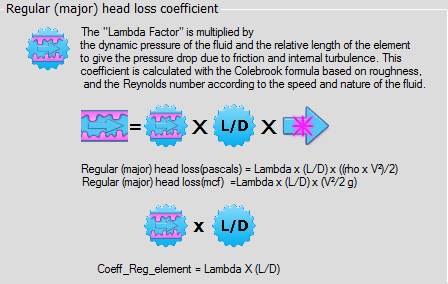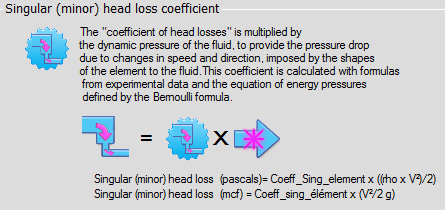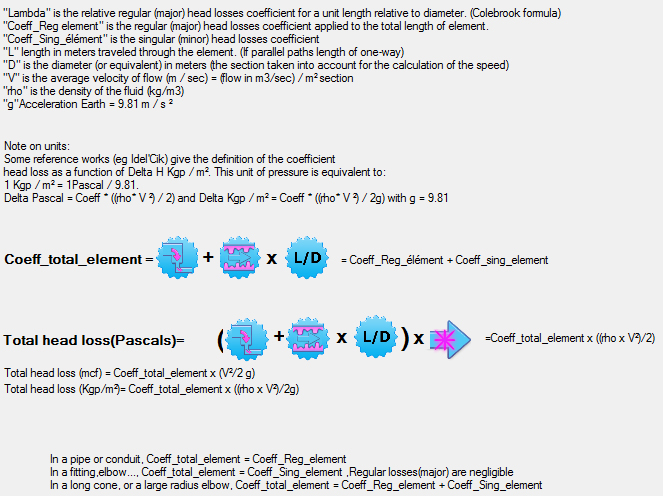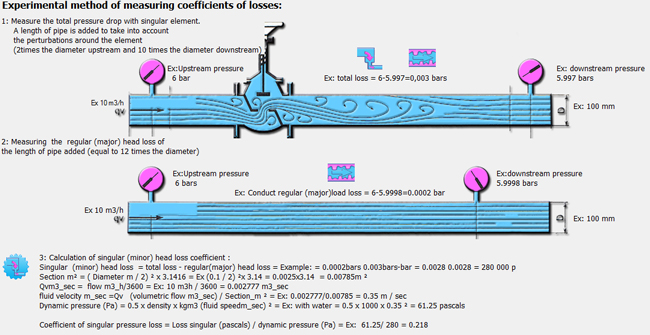Definition pressure drops head loss air water hydraulic aeraulic:

The losses in the pipes and networks aeraulic or hydraulic (air water gas) designates the irreversible loss of pressure energy, that undergoes a liquid or a gas as it passes through a conduit, or another fitting of fluid network .

these energy losses related to the fluid velocity (low speed = low pressure drop), is caused by the conversion into heat of the internal friction caused by:

• the viscosity of the fluid (a perfect fluid, without viscosity, does not generate a pressure drop),
• the roughness of the walls,
• the speed variations
• and changes in direction of the fluid.

L'unité de la perte de charge est une pression (pascals,bars...)The unit pressure drop is a pressure (Pascal, bars ...) or a height of water column that produces a hydrostatic head (head loss) (hydrostatic pressure) equivalent.The term "head loss" therefore means "loss of hydrostatic head." From this definition we can already say that the losses in the networks are important if:

• The fluid velocity is high and the roughness is important.
• Speed ​​variation due to the change of section is important and sudden.
• the change of direction is important and sudden.These energy losses are therefore minimum if:
• the speed is low and surfaces are smooth.
• the speed variation due to a change in section is gradual and low.
• the change of direction is low and progressive.• There are 2 types of pressure losses:
1.The major head loss , representing frictional head loss in the pipes. They are caused by the fluid viscosity.They depend on the degree of turbulence (described by the Reynolds number)..
2.The minor head loss, are the result of changes in speed and direction changes of the fluid caused by the shapes and obstacles encountered by the fluid passing through an object: Cones, elbows, grids, racordements, junctions ...

In reality, these two types of pressure losses may not be separate, and in a rounded elbow, it is some minor head loss due to change of direction and a part of major load loss due to friction along the length of conduit formed by the elbow. An addition of the two pressure losses may be necessary if the friction surfaces are important (a coil constituted of elbows) But usually, the major head loss are Neglected for fittings or other networks elements.

The pressure drop coefficient is a unitless value which calculates the pressure drop as a function of the dynamic pressure of the fluid.

•Dynamic pressure= 0.5 x Density(kg/m3) x Velocity²(m/sec)

Since there are two types of losses, there are two types of coefficients head loss:

1.Major head loss coefficient.
2.Minor head loss coefficient.There are various formulas to determine themajor loss coefficient ,The choice of formula depends on the flow regimewhich is estimated with the Reynolds number.A description of the method of calculation of the coefficient of head loss based on a measure of loss (Image taken from pro3D mecaflux software)